# Gauss-Manin connection

(Redirected from Gauss–Manin connection)

The Gauss–Manin connection is a way to differentiate cohomology classes with respect to parameters. Consider a smooth projective curveover a field. Its first de Rham cohomology group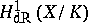can be identified with the space of differentials of second kind onmodulo exact differentials (cf. Differential). Each derivationof(cf. Derivation in a ring) can be lifted in a canonical way to a mapping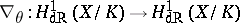satisfyingfor,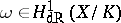[a1], [a2]. This amounts to a connection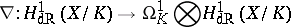which is integrable (i.e.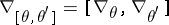). Ifis a function field in one variable, one obtains the Picard–Fuchs equation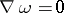, which has regular singular points (cf. Regular singular point).

The generalization to higher dimension is due to A. Grothendieck [a3]. For a proper and smooth morphismof-schemes the de Rham cohomology of the fibres ofis described by the locally free-modules, the relative de Rham cohomology sheaves. From now on suppose thatis of finite type overand let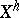and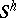denote the underlying analytic spaces. Thenand the analytic version of the Gauss–Manin connection is defined by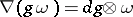for(respectively,) a local section of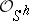(respectively,).

An algebraic construction has been given by N.M. Katz and T. Oda [a4]. The complex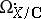is filtered by subcomplexes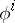, where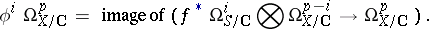One hasand. The connecting homomorphismin the long exact hypercohomology sequence associated to the exact sequence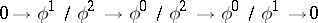is an algebraic version of the Gauss–Manin connection.

The Gauss–Manin connection is regular singular [a5][a8]. Its monodromy transformations around points at infinity are quasi-unipotent [a6], [a9], [a10], and bounds on the size of its Jordan blocks are known [a7], [a11]. Geometrical proofs of the monodromy theorem are due to A. Landman [a12], C.H. Clemens [a13] and D.T. Lê [a14].

Another important feature of the Gauss–Manin connection is Griffiths' transversality. The relative de Rham cohomology sheaves of a smooth proper morphism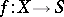can be filtered as follows. Let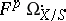be the subcomplex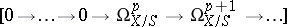of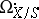. Then. The spectral sequencedegenerates at[a15] and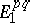is locally free on. Hence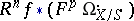maps injectively to a subsheaf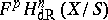of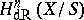. Griffiths' transversality is the property thatThe geometric data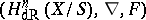have given rise to the concept of a (polarized) variation of Hodge structure. A. Borel has extended the monodromy theorem to this abstract case ([a16], (6.1)).

The Gauss–Manin connection has also been defined for function germs with isolated singularity [a10] and for mapping germs defining isolated complete intersection singularities [a17]. The monodromy of these connections is the classical Picard–Lefschetz monodromy on the vanishing cohomology.

In the theory of-modules (cf.-module), the theory of the Gauss–Manin connection is expressed as a property of the direct image functor for a proper morphism. Combined with the formalism of vanishing cycle functors [a18] it gives rise to the notion of the Gauss–Manin system [a19]. This plays an important role in the asymptotic Hodge theory of singularities [a20][a22].